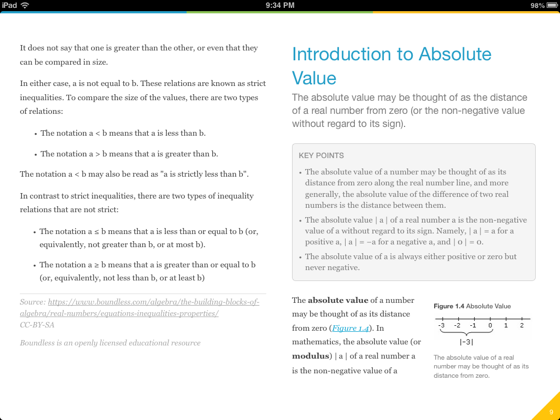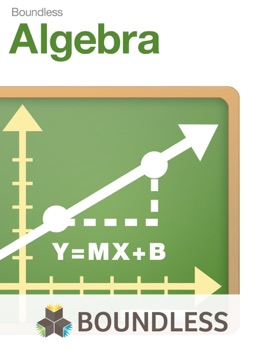• £5.99

## Publisher Description

Introduction to Algebra is a college-level, introductory textbook that covers the important subject of Algebra -- one of the basic building blocks of studies in higher mathematics. Boundless works with subject matter experts to select the best open educational resources available on the web, review the content for quality, and create introductory, college-level textbooks designed to meet the study needs of university students.

This textbook covers:

The Building Blocks of Algebra -- Real Numbers, Exponents, Scientific Notation, Order of Operations, Working with Polynomials, Factoring, Rational Expressions, Radical Notation and Exponents, Basics of Equation Solving

Graphs, Functions, and Models -- Introduction to Graphing, Functions: An Introduction, Modeling Equations of Lines, Functions Revisited, Algebra of Functions, Transformations

Functions, Equations, and Inequalities -- Linear Equations and Functions, Complex Numbers, Quadratic Equations, Functions, and Applications, Graphs of Quadratic Functions, Further Equation Solving, Working with Linear Inequalities

Polynomial and Rational Functions -- Polynomial Functions and Models, Graphing Polynomial Functions, Polynomial Division; The Remainder and Factor Theorems, Zeroes of Polynomial Functions and Their Theorems, Rational Functions, Inequalities, Variation and Problem Solving

Exponents and Logarithms -- Inverse Functions, Graphing Exponential Functions, Graphing Logarithmic Functions, Properties of Logarithmic Functions, Growth and Decay; Compound Interest

Systems of Equations and Matrices -- Systems of Equations in Two Variables, Systems of Equations in Three Variables, Matrices, Matrix Operations, Inverses of Matrices, Determinants and Cramer's Rule, Systems of Inequalities and Linear Programming, Partial Fractions

Conic Sections -- The Parabola, The Circle and the Ellipse, The Hyperbola, Nonlinear Systems of Equations and Inequalities

Sequences, Series and Combinatorics -- Sequences and Series, Arithmetic Sequences and Series, Geometric Sequences and Series, Mathematical Inductions, Combinatorics, The Binomial Theorem, Probability

•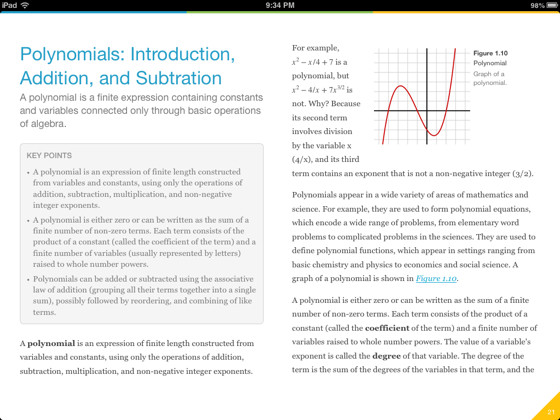•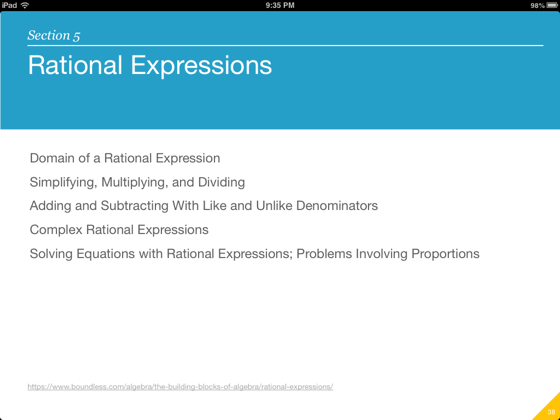•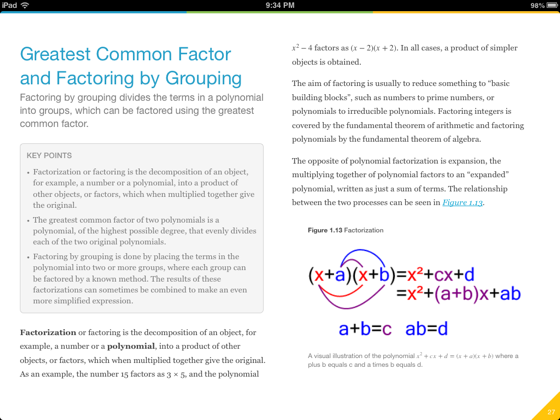•